# Division by zero

Mazurek, Leszek (2020) Division by zero. [Preprint]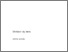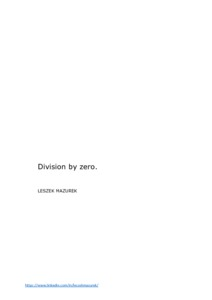Preview
Text
Leszek Mazurek - Division by Zero.pdf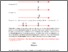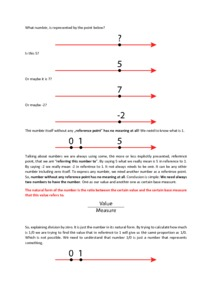Preview
Text
Leszek Mazurek - Short introduction to Division by zero.pdf

## Abstract

This paper solves the problem of division by zero. Starting with a deep analysis of multiplication and division, it is presented that they are one in the same, as an operation. Together with the operation of selection, they are different forms of the transformation operation, that changes one pair of numbers into another pair of numbers. It is presented that the numbers are always in reference to some other numbers. Therefore, the only correct form of the number, is when it is a ratio between the certain value and the base measure, which this value is related to. It is clearly proven that the problem of division by zero is the result of unauthorized simplification, which was done by bringing all the rational numbers (which are represented by the ratio of the value to the measure) to the fractions, with a denominator of 1. This paper shows why we should not make fractions with different numerators and denominators equal, even so they seem to be in the same proportion. Finally, there are two graphs with the functions presented 1/x and tg(x) with their discontinuity points, which are visible only on the real numbers graph. This result is from projecting points of the rational numbers graph onto the real numbers graph with a denominator of 1. These discontinuity points disappear when we present our graphs in rational numbers. This paper fixes one of the foundations of mathematics and shows us how to divide by zero. This is only the first issue that was solved, but based on the proper understanding of numbers in their natural form, still many other things need to be fixed. It is still difficult to predict all the consequences, which this change can bring, to everything that was created based on this wrong foundation.

 Export/Citation: EndNote | BibTeX | Dublin Core | ASCII/Text Citation (Chicago) | HTML Citation | OpenURL
 Social Networking:

Item Type: Preprint
Creators:
CreatorsEmailORCID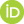Mazurek, Leszekleszek.mazurek@op.pl0000-0001-7481-9375
Additional Information: Short introduction here: https://vixra.org/abs/2002.0070
Keywords: Division by zero, Real numbers, Rational numbers, Ratio, Fraction, Fixing mathematics, Numbers
Subjects: Specific Sciences > Mathematics > Explanation
Specific Sciences > Mathematics > Foundations
Specific Sciences > Mathematics > Logic
Specific Sciences > Mathematics > Proof
General Issues > Explanation
General Issues > History of Philosophy of Science
Specific Sciences > Mathematics
General Issues > Science Education
General Issues > Theory Change
General Issues > Values In Science
Depositing User: Mr. Leszek Mazurek
Date Deposited: 16 Feb 2020 19:34
Item ID: 16915
Official URL: https://vixra.org/abs/2001.0475
DOI or Unique Handle: 10.5281/zenodo.3668512
Subjects: Specific Sciences > Mathematics > Explanation
Specific Sciences > Mathematics > Foundations
Specific Sciences > Mathematics > Logic
Specific Sciences > Mathematics > Proof
General Issues > Explanation
General Issues > History of Philosophy of Science
Specific Sciences > Mathematics
General Issues > Science Education
General Issues > Theory Change
General Issues > Values In Science
Date: 22 January 2020
URI: http://philsci-archive.pitt.edu/id/eprint/16915

### Monthly Views for the past 3 yearsView Item